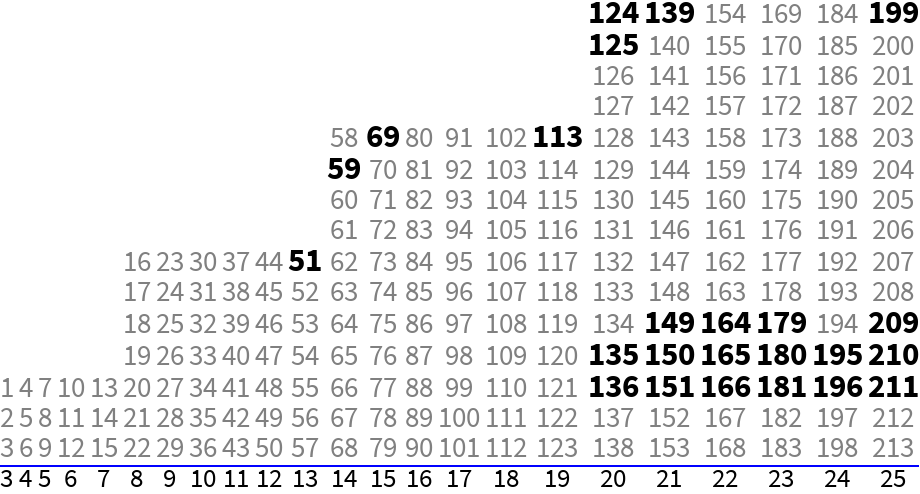#Function Repository Resource:

# ReasonableRuler

Find a near minimal set of marks for an integer length ruler so that all smaller integer distances are measurable

Contributed by: Ed Pegg Jr
 ResourceFunction["ReasonableRuler"][length] finds a set of integers with differences giving all values up to length using a compact form. ResourceFunction["ReasonableRuler"][length,"Full"] finds a reasonable ruler for given length, showing the full set of values.

## Details and Options

The "Full" calculation requires significant memory for large length values.

## Examples

### Basic Examples

Marks for a sparse ruler of length 57:

 In:=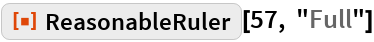Out=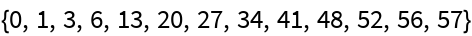Check that all differences are covered:

 In:=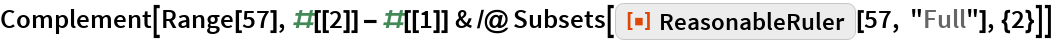Out=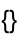Show spaces between marks in a the full form of a length 396 ruler:

 In:=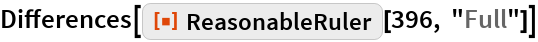Out=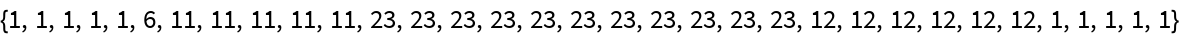Split the Difference:

 In:=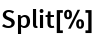Out=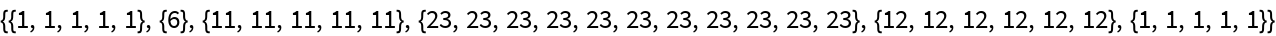Make a series of lists from the compact form of a length 396 ruler:

 In:=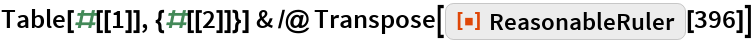Out=Show the compact form:

 In:=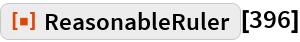Out=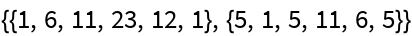Verify the above shortened form has length 396:

 In:=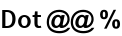Out=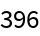Show the full form for a length 396 ruler:

 In:=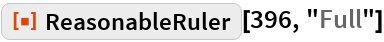Out=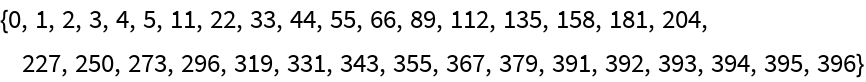### Scope

Count the number of marks on a length 58 ruler:

 In:=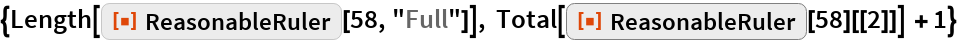Out=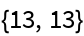The excess of a ruler with length L and M marks is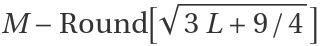:

 In:=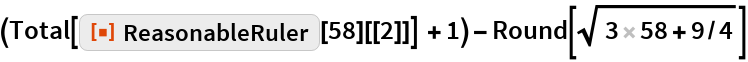Out=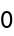A reasonable ruler has an excess of 0 or 1:

 In:=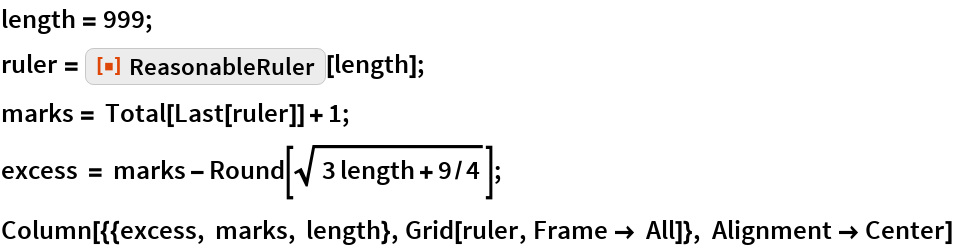Out=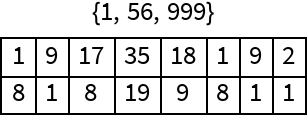A list of some rulers with a single mark less than those generated by this function:

 In:=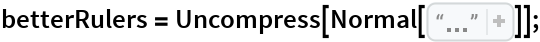Show the length 999 ruler with one fewer marks:

 In:=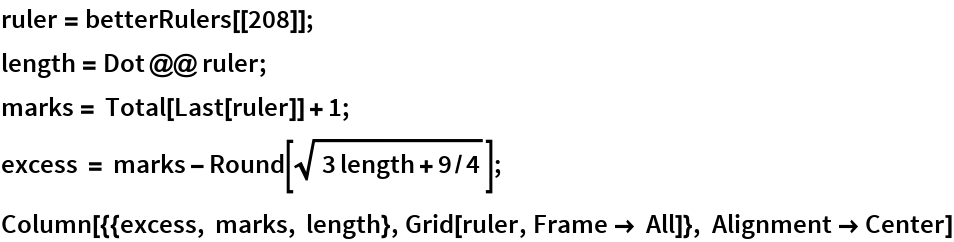Out=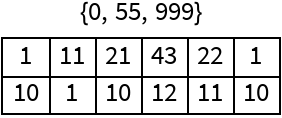Show how the John Leech upper bound for the number of marks in a complete ruler compares to function-generated rulers:

 In:=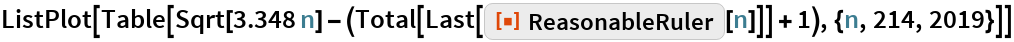Out=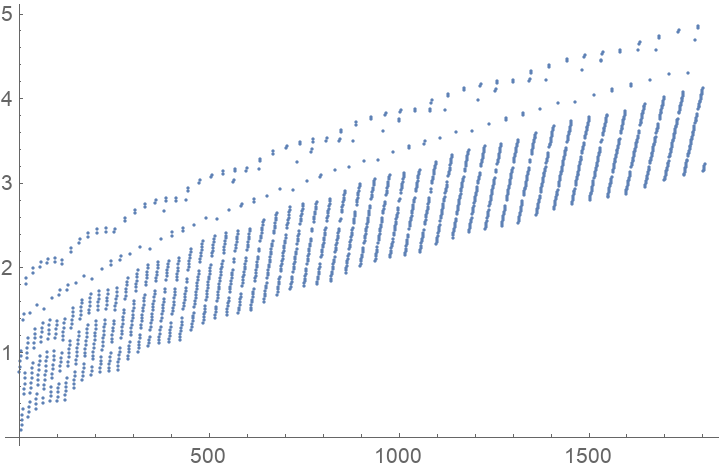Generate rulers of length n! and plot the number of marks:

 In:=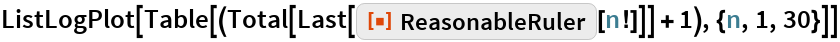Out=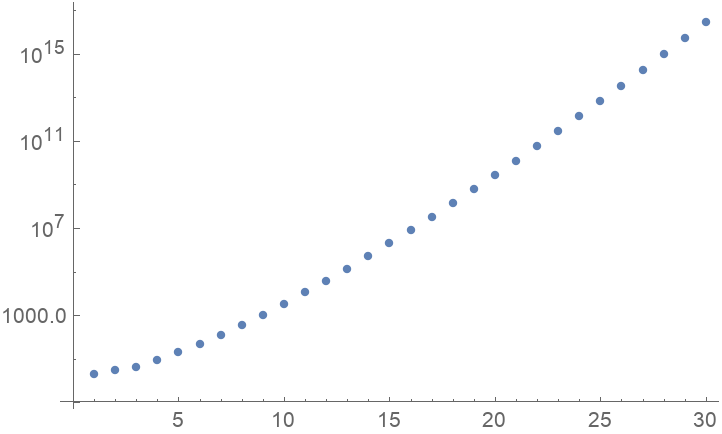### Options

Show the marks for the first 51 rulers:

 In:=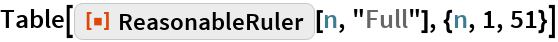Out=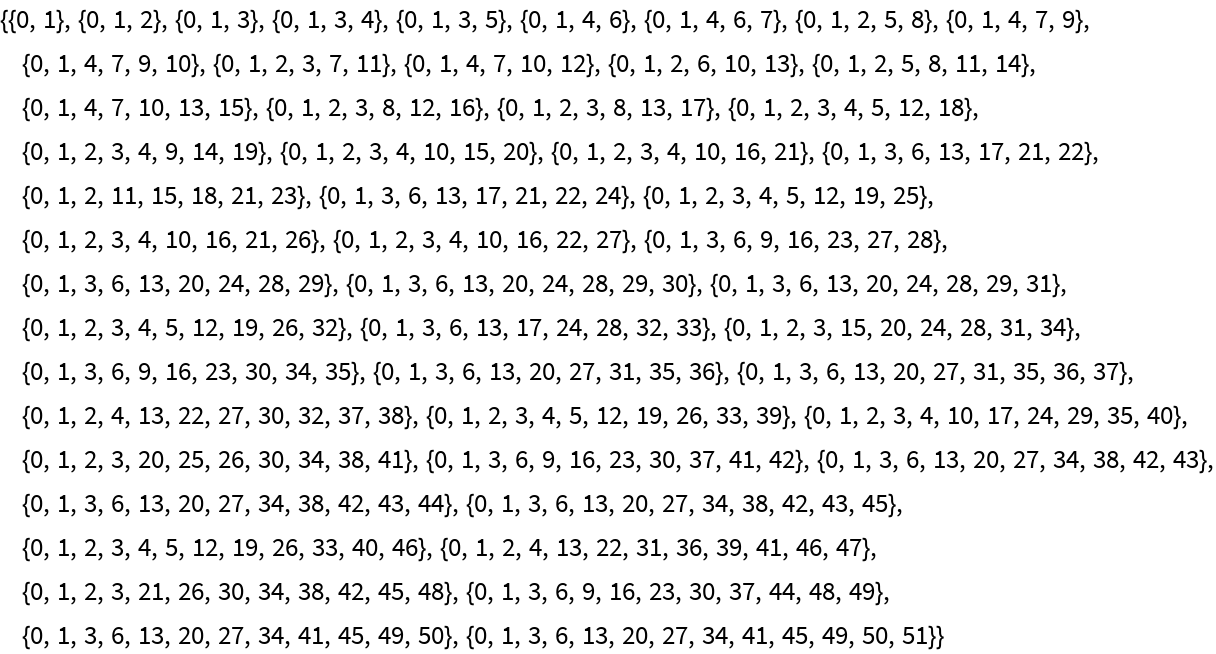Show the excess values for the first 51 rulers:

 In:=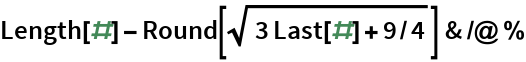Out=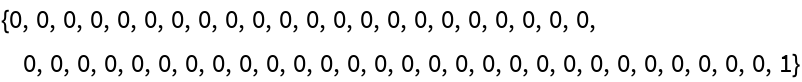### Possible Issues

Show the default shortened form for a length googol ruler:

 In:=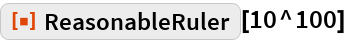Out=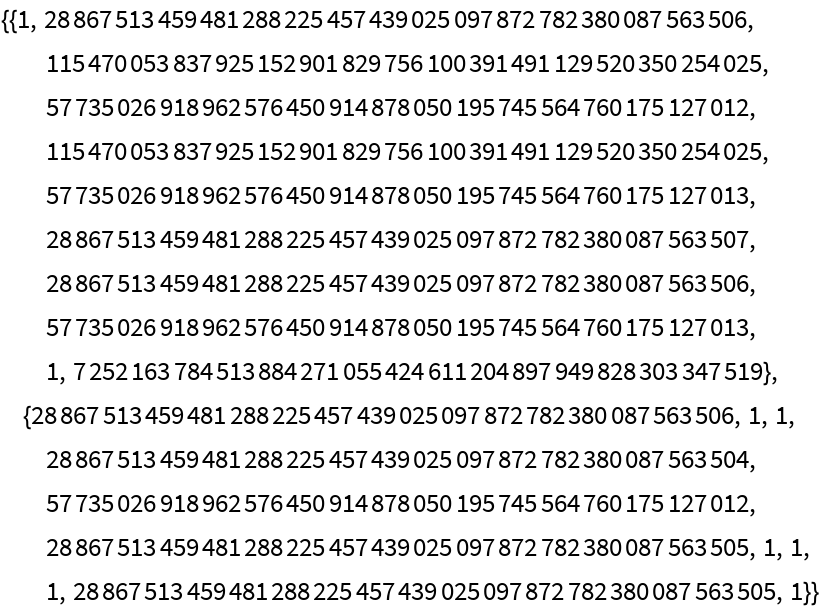Show the number of marks in a length googol ruler:

 In:=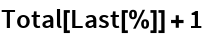Out=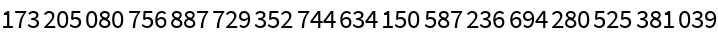Showing the full form of a googol-length ruler isn’t recommended.

### Neat Examples

Generate reasonable rulers for various integer powers and calculate their excess:

 In:=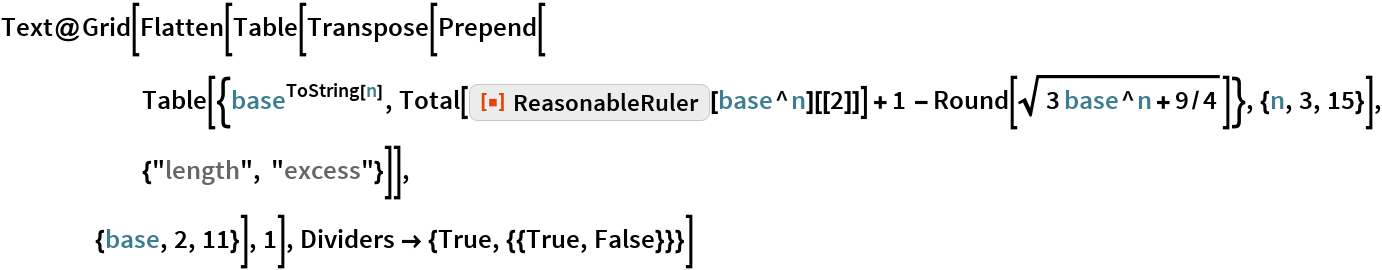Out=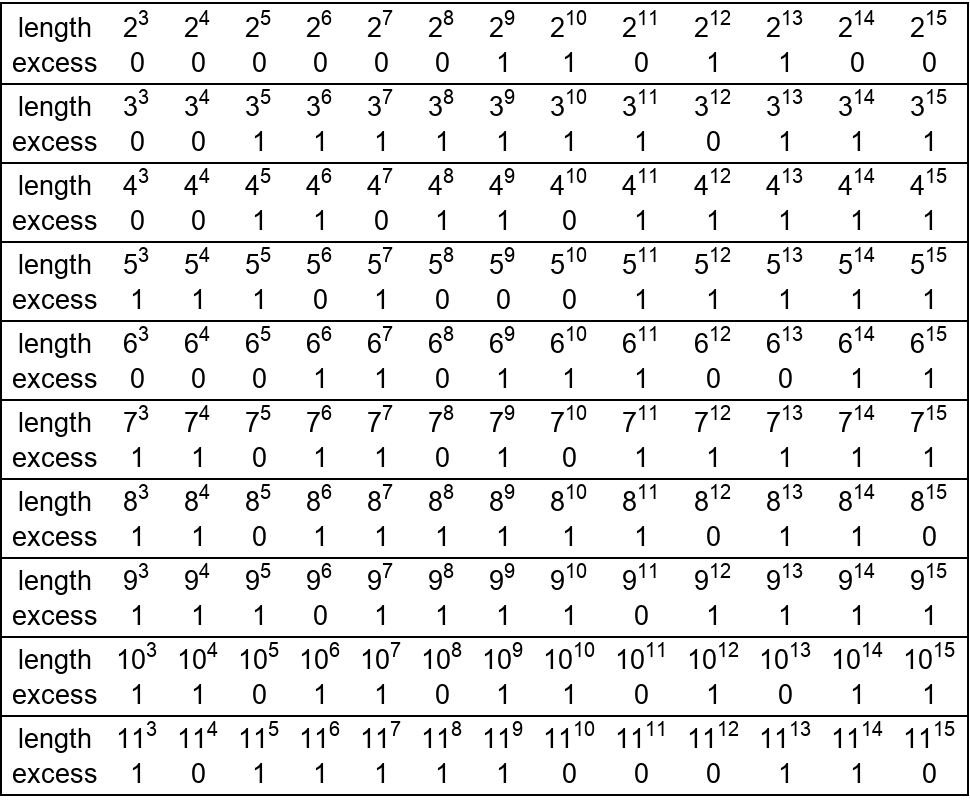All rulers to length 213 are minimal. Show a pixel representation of these sparse rulers:

 In:=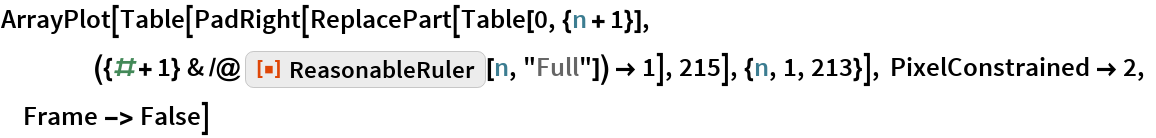Out=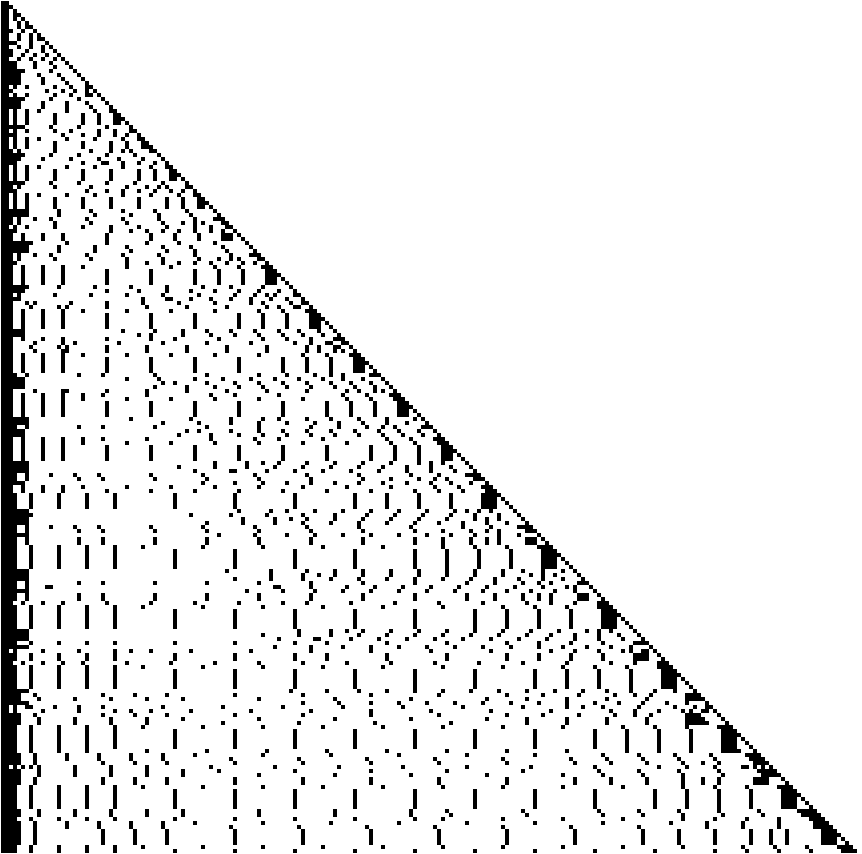The maximal length for a given number of marks is usually a Wichmann value:

 In:=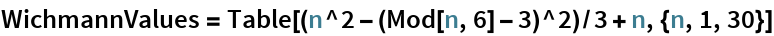Out=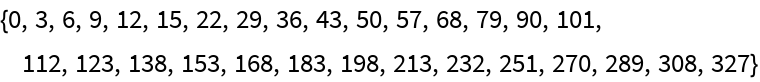Arrange lengths in columns ending in Wichmann values and bold the lengths with excess 1:

 In:=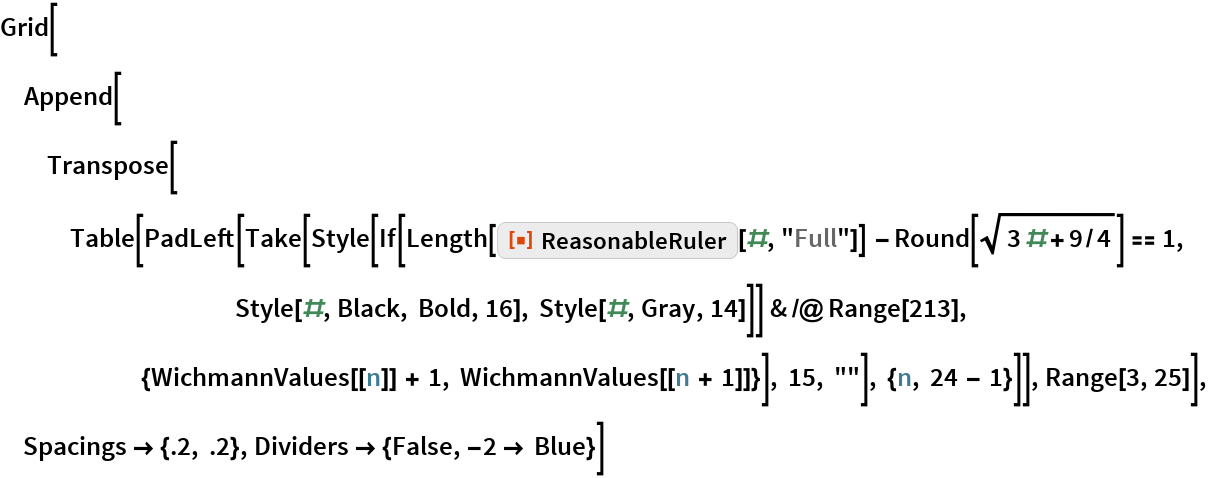Out=